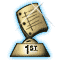Home >> A Tsl >> Archives >> 00 1 >> As Easy As Pi!

## Search form

 Home > Teacher Lesson Plans > Archives > Lesson Plan L E S S O N     P L A N As our highlighted lesson, the submitter was awarded a \$50 honorarium. See our guidelines to submit yours!As Easy As Pi! Subject: Geometry, Measurement Grade: 6-8 Brief Description Students conduct a scavenger hunt to find circular objects at home, then use the objects to find the pattern of pi. Objectives Students use and explain pi and the formula for circumference. Keywords pi, circumference, diameter, measurement, geometry, decimals, division Materials Needed string rulers calculators student-selected circular objects paper pens or pencils chalkboard chalk Lesson Plan Organize students into groups and ask each group to bring in a variety of items that are circular in shape, such as a soup can, a frying pan lid, a Frisbee, an ice-cream container. Discuss the terms center point, circumference, radius, and diameter with students. Help students see the relationship between radius and diameter by having them write a formula that could be used to find diameter using the radius (diameter of a circle = 2 x radius). Help students understand that as circumference increases so does diameter. Tell students that they will find a pattern in the measurements' relationship to each another. Brainstorm strategies for measuring circumference and diameter. Review measuring with centimeters to the nearest millimeter. Tell students to find the circumference and diameter of at least five objects of their choice, measuring to the nearest millimeter. On their papers, students should divide the circumference by the diameter and round to the nearest 100th. Have each group record their information on a chart on the chalkboard. Use the chart to look for a pattern. As students begin to see that circumference is always a little more than three times the diameter, introduce pi. Tell students that pi is about 3.14. Discuss why their numbers may not represent exact measurements. Ask students to look at their calculators to see whether they have the pi button. If they do, have them press that button. (Usually the calculator goes to the hundred-thousandths place.) Discuss why pi continues on and on. Ask students to identify how pi might help them find the circumference of a circle. Guide them to write the formula. Then have them use the formula to find the circumference of their circular objects. ( Pi = 3.14. The circumference of a circle = pi x diameter. The diameter of a circle = 2 x radius.) Assessment After the discussion, assess learning by asking students to write about the relationship of circumference, diameter, radius, and pi in their journals. Have them show an example of how to use the formula. Lesson Plan Source Submitted By Brenda Lium, Agassiz Middle School, Fargo, North DakotaAs our highlighted lesson, the submitter was awarded a \$50 honorarium. See our guidelines to submit yours! 04/04/2001 In an effort to keep our Lesson Plan Database as current as possible, please email us to report any links that are not working.